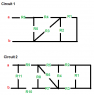# Finding equivalent resistance for the given circuits

• Engineering
• 24karatbear
In summary, the homework equation for circuits 1 and 2 is to combine resistors in parallel and in series, respectively.

## Homework Statement

Find the equivalent resistance Rab for each circuit given here (resistance values have been omitted so I can focus on understanding the concept, but I can supply them if requested).

## Homework Equations

This problem expects me to know how to combine resistors in series/parallel.

Series: Req = R1 + R2 + ... + Rk
Parallel: Req-1 = R1-1 + R2-1 + ... + Rk-1

## The Attempt at a Solution

Attempt for circuit 1:
- Combine R1 and R2 in parallel.
- Combine R3 and above Req in parallel.
- Combine R4 and above Req in series.
- Combine R5 and above Req in parallel.
- Combine R6 and above Req in series.

Attempt for circuit 2:
Here is where I had a lot of trouble. The conductor at the right end of the circuit is what's throwing me off. I reconstructed this circuit in a simulator and it was showing that the voltage across R1 is 0, but I'm not sure why. Also, I don't know how to start combining them. R2 and R3 aren't in parallel (at least, I don't think they are), and I don't think R1, R2, and R3 are in series since the current across R1 would be different than the current across R2 and R3. Any push in the right direction would be greatly appreciated!

#### Attachments

•circuitdiagrams.png
3.6 KB · Views: 587
Your method for circuit 1 looks fine.

24karatbear said:
Attempt for circuit 2:
Here is where I had a lot of trouble. The conductor at the right end of the circuit is what's throwing me off. I reconstructed this circuit in a simulator and it was showing that the voltage across R1 is 0, but I'm not sure why. Also, I don't know how to start combining them. R2 and R3 aren't in parallel (at least, I don't think they are), and I don't think R1, R2, and R3 are in series since the current across R1 would be different than the current across R2 and R3. Any push in the right direction would be greatly appreciated!

Think of the wire in parallel with R1 as a resistance of zero Ohms. What do you get when you place any resistance R in parallel with a resistance of zero?

gneill said:
Your method for circuit 1 looks fine.

Think of the wire in parallel with R1 as a resistance of zero Ohms. What do you get when you place any resistance R in parallel with a resistance of zero?

Thank you for having a look! I discovered that it is a short, and therefore all the current is going through it. Because of this, the voltage across R1 is zero, adn there is zero current going through R1. This means that R2 and R3 can be taken in series. Would this be correct?

24karatbear said:
Thank you for having a look! I discovered that it is a short, and therefore all the current is going through it. Because of this, the voltage across R1 is zero, adn there is zero current going through R1. This means that R2 and R3 can be taken in series. Would this be correct?
That would be correct; R1 effectively disappears from the circuit, replaced by the wire.

•24karatbear
gneill said:
That would be correct; R1 effectively disappears from the circuit, replaced by the wire.

Okay, great! Thanks again for looking over my post, gneill. :)